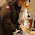## Monday, April 16, 2012

### Why to use highest density intervals instead of equal tailed intervals

I recently received this question in an e-mail:

We've obtained a dataset that reports hospital rates of hospital-acquired bed sores (as % of patients, based on hospital-wide patient exams 4 days per year), and they also provide CI's for these point estimates "based on Bayesian statistics" (that's all we know, we're not given any more info)….and I'm confused because many of the hospitals that had a rate of zero events are paired with a confidence interval that doesn't contain zero (confidence interval is higher than zero). What do you think about that?

If they really are using Bayesian "confidence intervals", and a frequency of zero does not produce a CI that includes zero, then I bet they are using equal-tailed credible intervals. By definition, a 95% equal tailed credible interval has to exclude 2.5% from each tail of the distribution. So, even if the mode of the posterior is at zero, if you exclude 2.5%, then you have to exclude zero. That's why I use highest density intervals (HDIs), not equal-tail CIs. HDIs always include the mode(s).

Here is an example of what I mean. Suppose there are zero "heads" in N=10 flips of coin, being modeled with a Bernoulli likelihood. Suppose we start with a uniform prior --- that is, dbeta(1,1) --- on the bias of the coin. Then the posterior is dbeta(1,11), which looks like this:

The 95% HDI goes from zero to 0.2384. But the 95% equal-tailed interval goes from 0.0023 to 0.2849, which excludes zero. Clearly the HDI seems to be a more intuitive and meaningful summary of the posterior.

There is a way that the posterior 95% HDI could exclude zero even when the data have a frequency of zero. It can happen if the prior already excludes zero. For example, the prior might be dbeta(1.01,1.01), or dbeta(2,10), or whatever.

And, of course, the analogous argument applies when the likelihood is Poisson and the prior is gamma, or what have you.

1.2.3.